# 1. 介紹

• 效能問題的發生時常是間歇性的，不容易掌握
• 沒有人對所有設備系統是有完整的控制權

NF chain 為 Firewall → VPN，當 VPN 有部分封包發生高延遲時，營運商可能會懷疑是 VPN 出問題，但到最後才發現移除掉 Firewall 就沒事了，問題原來是出在 Firewall。 有時候突發流量（bursty traffic）會造成 Firewall 的佇列（queue）產生，進而導致高延遲發生。但這次原因不是這個，而是 Firewall 有個 bug 導致處理時間大幅增加！

• 錯誤配置、bugs
• 異常流量
• 系統中斷（interrupt）
• 負載平衡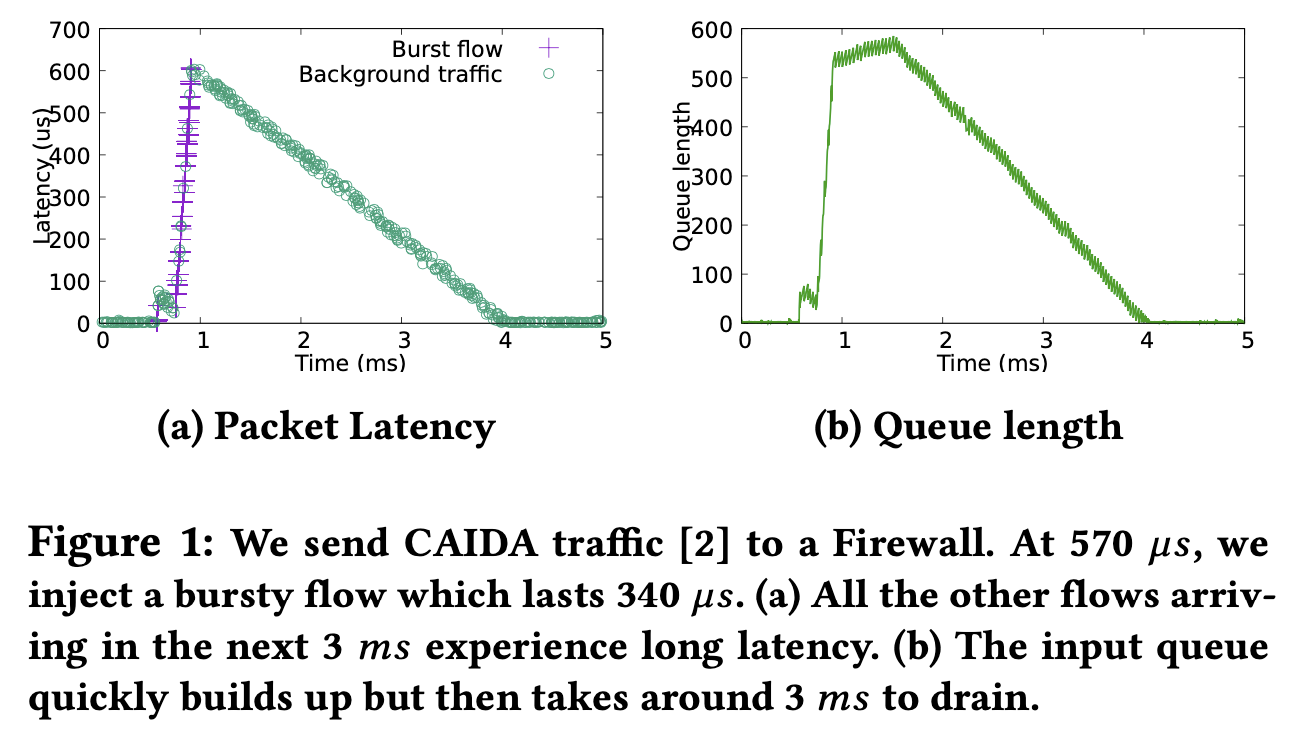Microscope 會直接針對每個 NF 的 queue 做監控側錄，並在離線時分析（因為會花很多時間），藉此找出問題的因果關係。

# 2. 動機

## 2.1 挑戰與 NF 診斷

Microsecond 等級的影響

§1 的圖片說明了：

• 資源競爭嚴重性
• 當前流量
• NF 的處理速率

• NF、流量源頭的異常
• Queue 的佔用率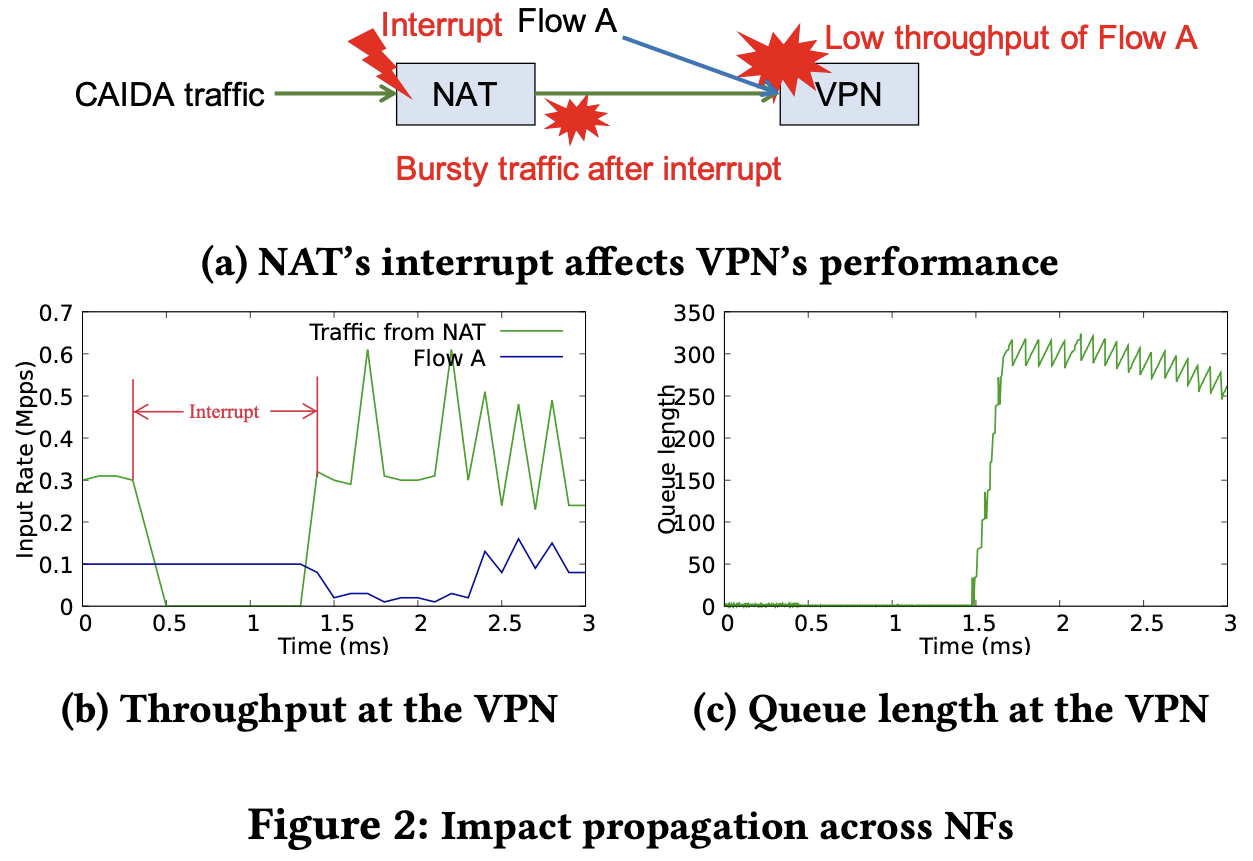• NAT 流量 0.25 Mpps
• Monitor 流量 0.05 Mpps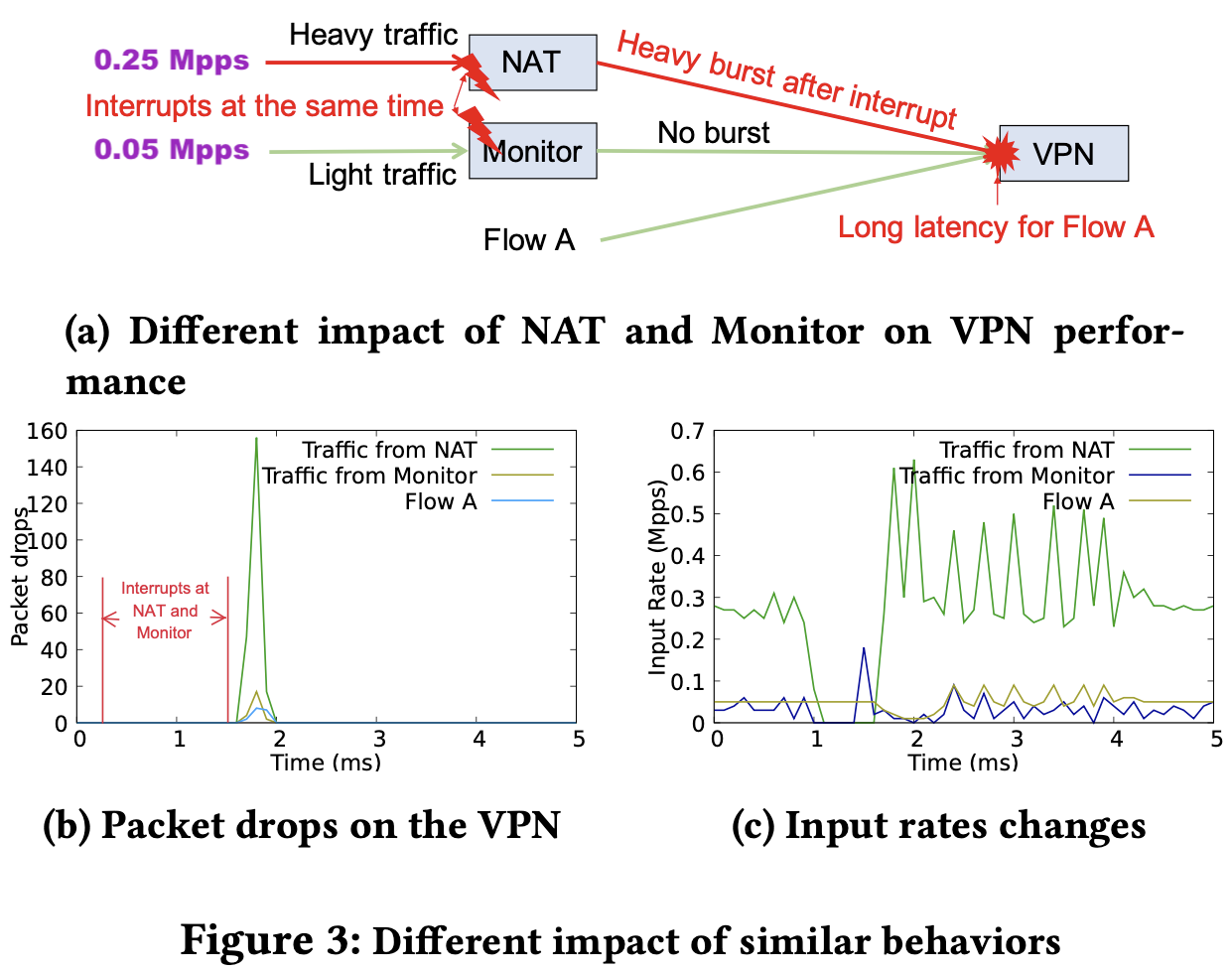## 2.2 效能診斷問卷

• 低吞吐量
• 間歇性發生的
• 單一使用者才有的問題

• 資源競爭（7 operators）
• traffic bursts（12 operators）
• interrupts（5 operators）
• NF bugs（15 operators）
• NF 相互影響
• 上游 NF 流量造成（6 operators）
• 錯誤配置（8 operators）
• 資源競爭（3 operators）

• Ranked list of root causes (12 operators)
• High accuracy (9 operators)
• Aggregated flows of each cause (7 operators)

# 3. 核心想法

• 進來的封包太多
• NF 處理太慢

$$\text {<culprit flow aggregates, culprit NF>} \rightarrow \text {<victim flow aggregate, victim NF>}: score$$

# 4. Microscope 設計

Microscope 僅收集每個封包的 timestamp、NF 間的 queuing 與 flow 狀態，而不存取 NF 內部程式碼。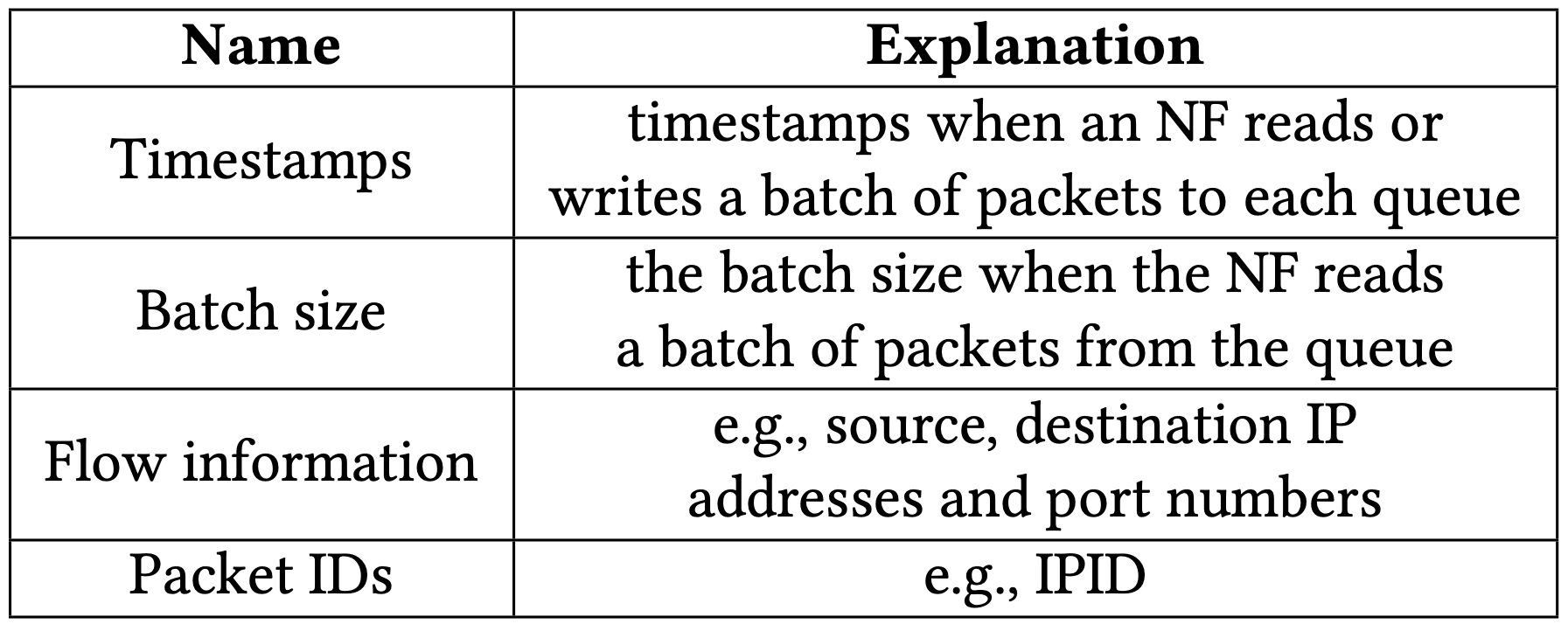1. 對 NF 中每個 victim packet（遭遇高延遲、低吞吐量、掉包）進行 local diagnosis，以確認原因是在 local 還是上游 NF。§4.1
• 觀察 queuing period，看問題是低處理量（local）還是 high input rate（上游 NFs）
2. 如果是因為 high input rate，那就進行 propagation analysis 以識別上游 NFs。§4.2
• 檢查 queuing period 的封包（也就是 Preset packets），並且分析每個 NF 中封包 timespan 的變化
3. 如果該 NF 造成了 high input rate，其也很可能遭受到了效能問題，因此進行 recursively diagnoses，也就是第 (1)、(2) 步。§4.3
4. 進行 aggregates causal relations，彙整並列出 culprit 到 victim 間的封包與 NF 資訊。§4.4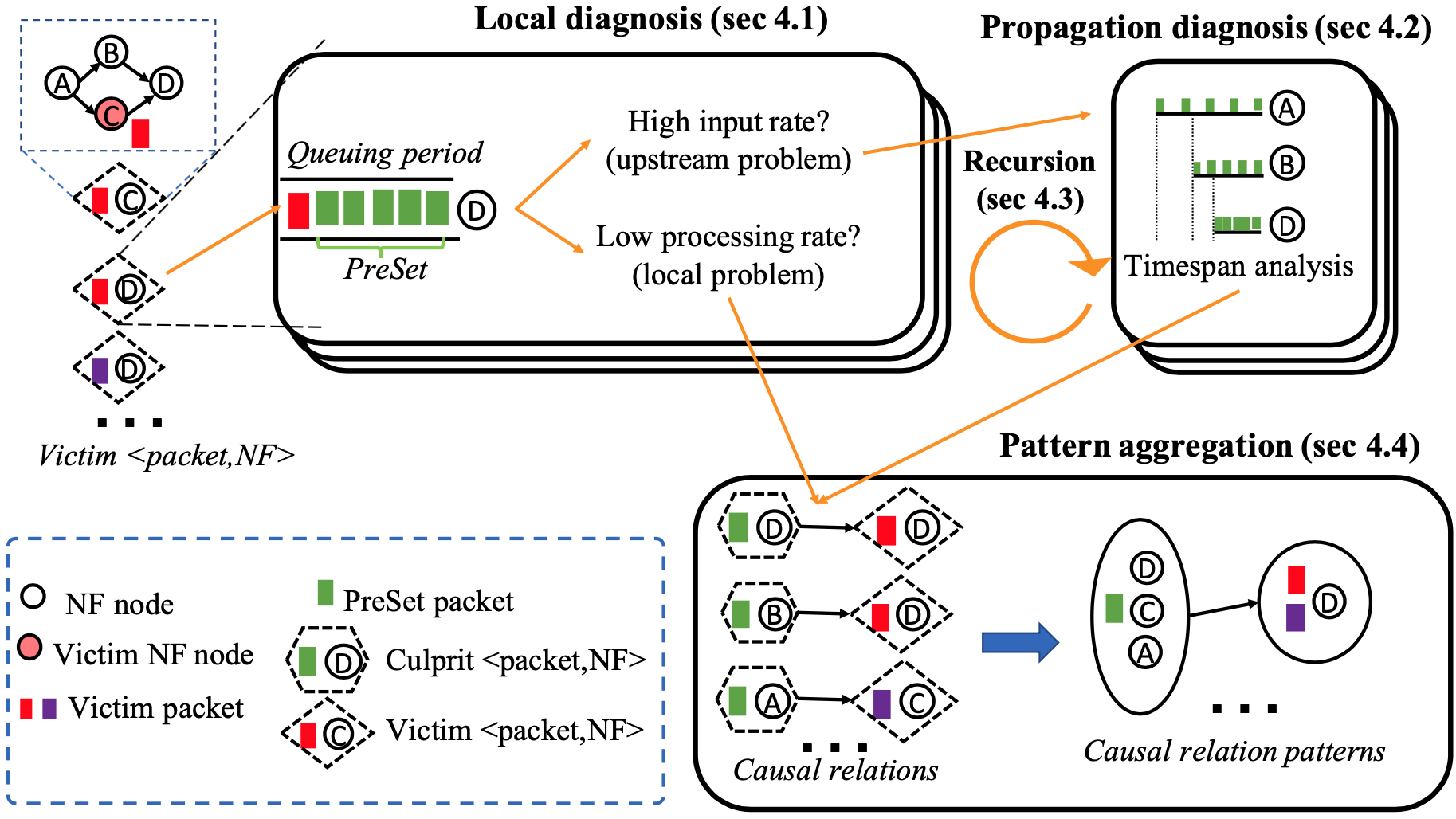## 4.1 Local Diagnosis

1. 大量的封包進入
2. 低落的封包處理效能

• $f$：NF
• $T$：某 NF 佇列出現的時間長度
• $n_i(T)$：在 $T$ 時間內到達的封包量
• $n_p(T)$：在 $T$ 時間內被處理的封包量
• $r_i$：NF 的峰值效能處理量（在相同軟硬體下）
• $S^f_i$：在最佳效能下（$r_i$）無法消化造成累積在佇列的封包量（輸入負載分數）

$$S_{i}^{f}=\left{\begin{array}{cl}n_{i}(T)-r_{i} \cdot T & \text { if } n_{i}(T) \geqslant r_{i} \cdot T \ 0 & \text { if } n_{i}(T)<r_{i} \cdot T\end{array}\right.$$

• $S^f_p$：實際處理的量比預期的少多少（處理分數）

$$S_{p}^{f}=\left{\begin{array}{ll}r_{i} \cdot T-n_{p}(T) & \text { if } n_{i}(T) \geqslant r_{i} \cdot T \ n_{i}(T)-n_{p}(T) & \text { if } n_{i}(T)<r_{i} \cdot T\end{array}\right.$$

$$S^f_i + S^f_p = n_i - n_p$$

## 4.2 Propagation Diagnosis

1. 上游 NF 忽然大幅增加封包處理量
2. 流量來源本身

• $PreSet(p)$：當封包 $p$ 到達 NF $f$ 時，其佇列已經持續了 $T$ 時間；在 $n_i(T)$ 累積的過程中，所有參與封包的統稱
• $timespan$ of $PreSet(p)$：在 $PreSet(p)$ 中「第一個」與「最後一個」離開該 NF 的封包時間差
• $T_{source}, T_{A}, T_{B}$：在 source、NF A、NF B 的 timespan

### 遍歷單條 VNF chain

• 在 A 遇到 interrupt 需等待，之後封包們緊湊（back-to-back）的被處理
• 封包間時間間隔被壓縮，相較於 $T_{source}$，$T_A$ 的 timespan 被壓縮
• 在 B 的處理時間較長，因此 $T_B$ 相較之下增加
• 在 C 遇到其他流量封包（cross traffic）的佇列需等待，因此 $Tc$ 被壓縮
• 這是變項對 $f$ 造成突發（bursty）封包，因為 $f$ 無法在 $T_C$ 時間內處理完，進而影響到封包 $p$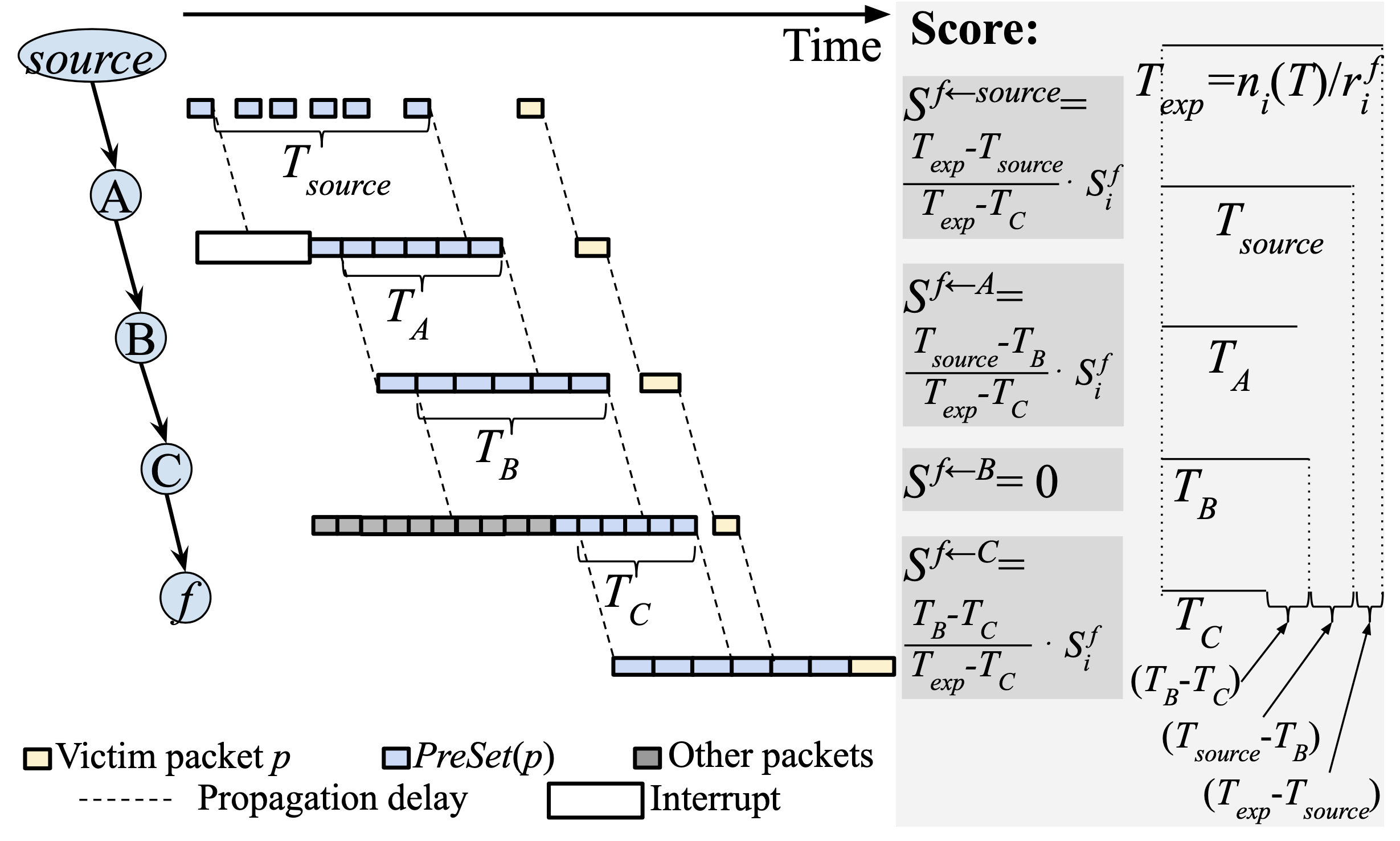• 對 C 而言，因為從 $T_B$ 減少了一些 timespan：

$$S^{f \leftarrow C} = \frac{T_B-T_C}{T_{exp}-T_C} \cdot S^f_i$$

• 對 source 而言，其減少了 $T_{exp} - T_{source}$
• （如果其值 > 0，表示從源頭開始就送的太急了）

$$S^{f \leftarrow source} = \frac{T_{exp} - T_{source}}{T_{exp}-T_C} \cdot S^f_i$$

• 對 A 而言，其減少了 $T_{source} - T_B$，因為 B 相對於 A 是增長的

$$S^{f \leftarrow A} = \frac{T_{source}-T_B}{T_{exp}-T_C}$$

• 對 B 而言，因為相對於 A 是增長的，因此肇責為 0

### 遍歷 DAG NF（多條路徑）

• $PreSetPath(p)$：從 source 到 $f$ 間是 DAG 的話，$PreSet(p)$ 所有經過路徑的集合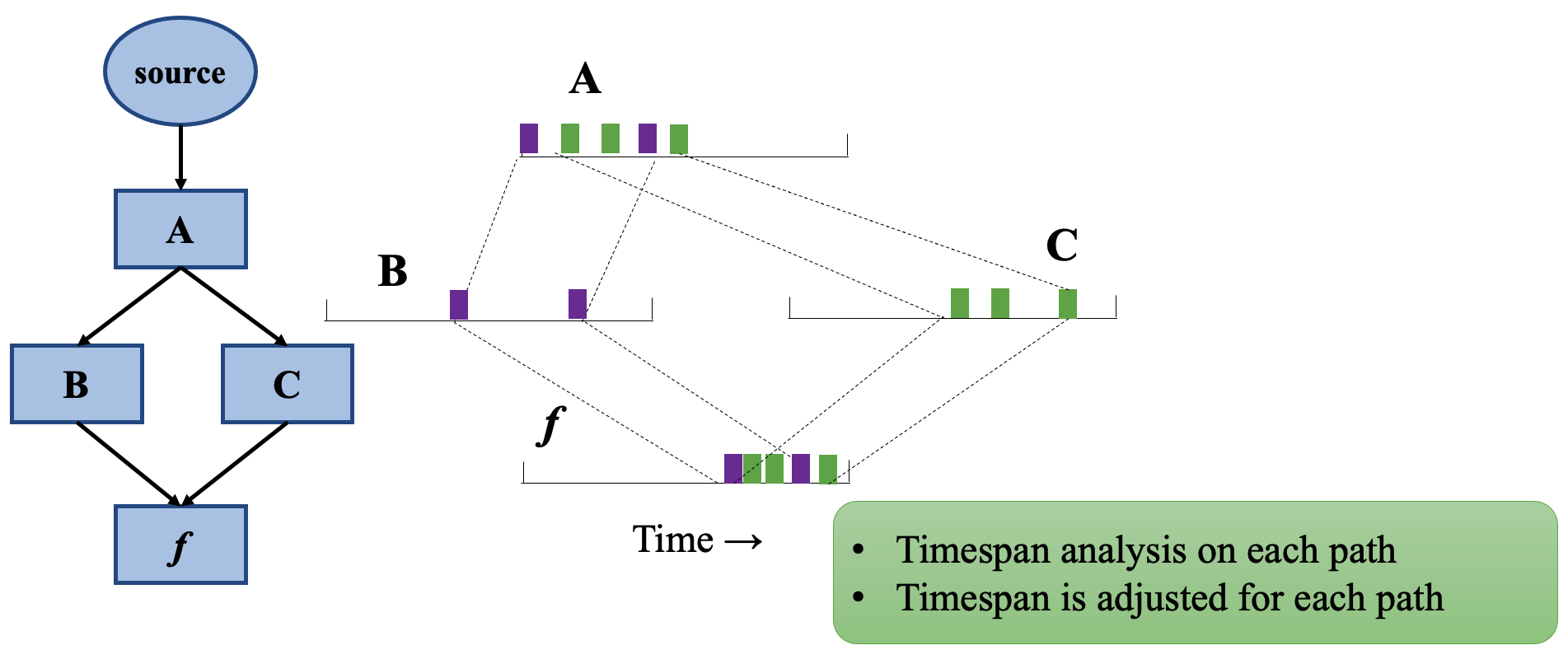• 當封包是完全交錯（fully interleave）

• 那麼 timespan 就會跟 $PreSet(p)$ 相同為 $n_i(T)/r^f_i = T_{exp}$
• 當封包不是完全交錯

• 那麼 timespan ≤ $T_{exp}$
• 也就代表或有一條或多條路徑較為突發（也就可能是戰犯）

編按：為何可能是戰犯？

• 單純的加總起來不可行
• 原因為多條路徑可能會聚在某個 NF 上，timespan 加總就很可能超過 $S^f_i$
• 按比例縮小所有分數

## 4.3 遞迴診斷 PreSet 封包

1. 到達 source
2. NF 的 $S_i$ 值都不是正的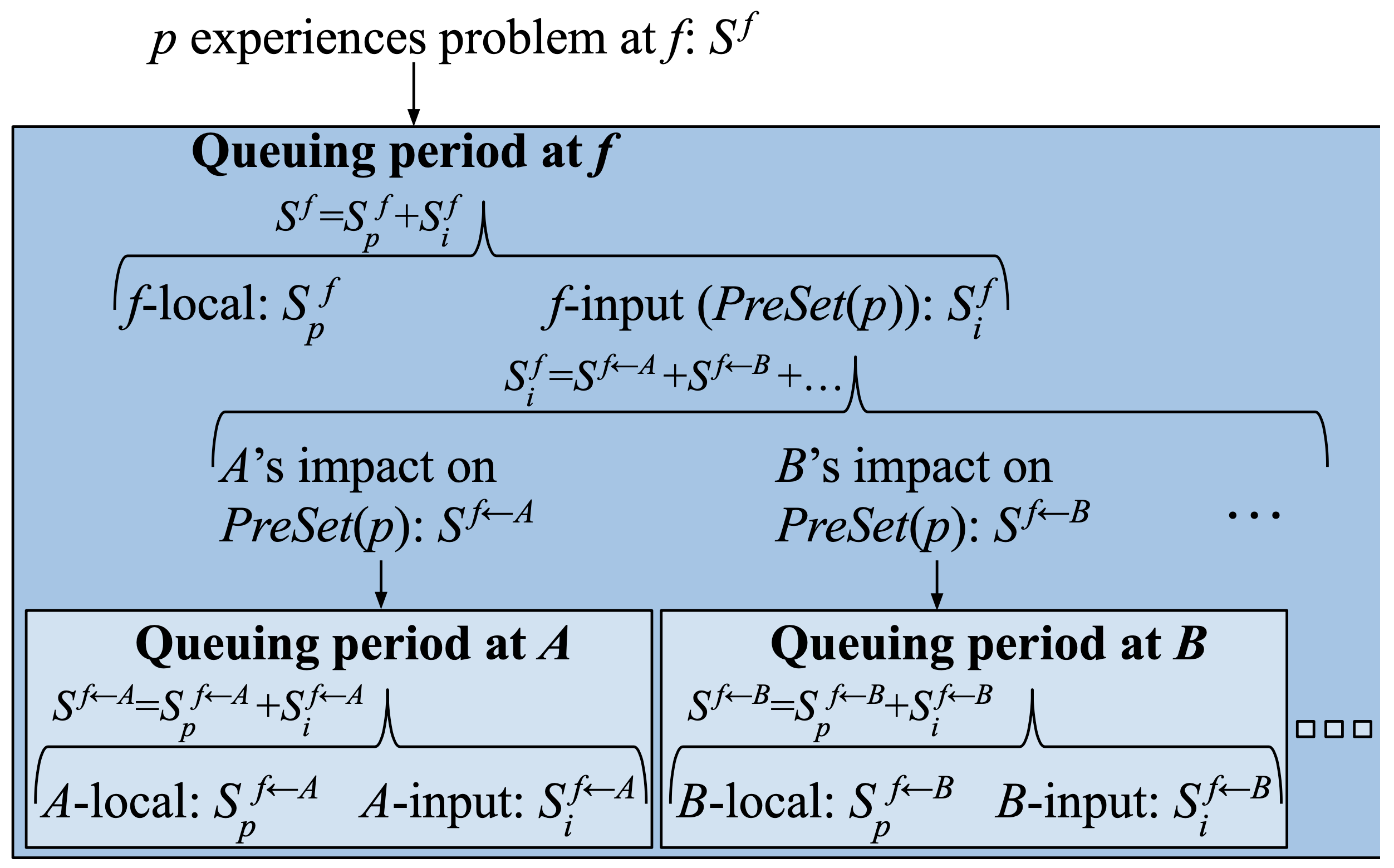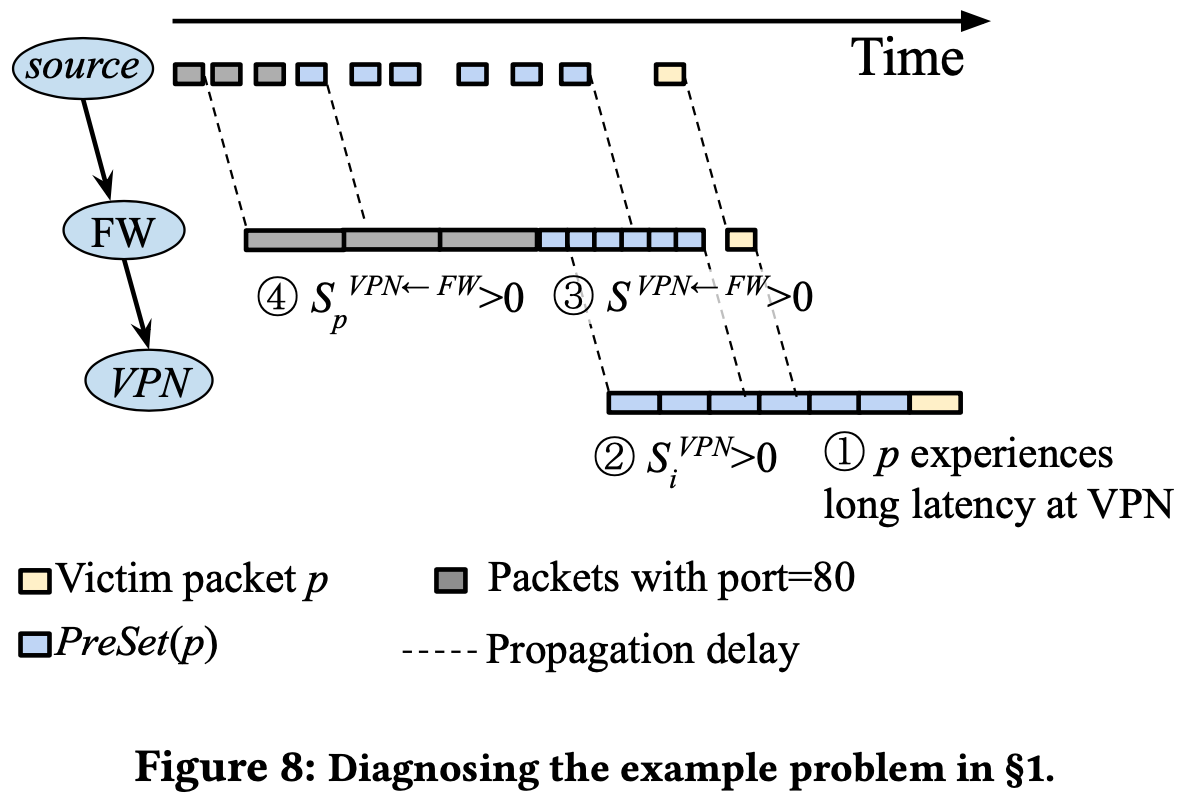## 4.4 Pattern Aggregation

$$\text {<culprit packets, culprit NF>} \rightarrow \text {<victim packet, victim NF>}: score$$

$$\text {<culprit flow aggregates, culprit NF set>} \rightarrow \text {<victim flow aggregates, victim NF set>}: score$$

• flow aggregates 包含
• source IP prefix
• source port range
• destination IP prefix
• destination port range
• protocol set
• NF set 包含
• NFs
• traffic sources

Pattern aggregation 演算法分為三步驟：

1. 將 culprit packets 與 culprit NF 分類。對每個 $\text {<culprit packet, culprit NF>}$，作者運行 AutoFocus 在 $\text {<victim packet, victim NF>}$ 上，產出一些有 victim packet/NF 欄位的 intermediate pattern aggregates。
2. 在 intermediate pattern aggregates 上再次運行 AutoFocus，以產生 final significant pattern aggregates。

# 5. 實作

Microscope 的組成是由：

• Data collector（執行階段）
• Diagnosis module（離線）

## 5.1 Runtime information collection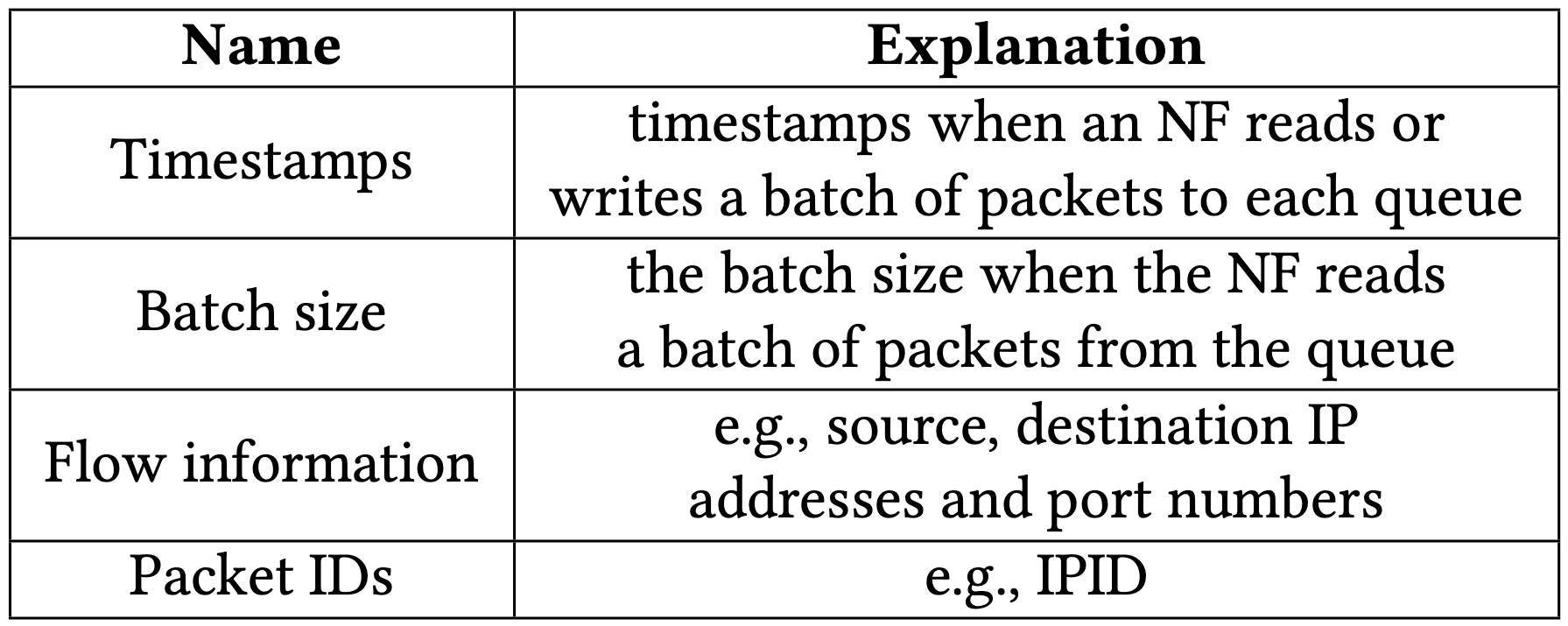• 每個封包都會記錄下這些資訊：
• five-tuple（source, destination 的 IP 與 port）
• IPID（可能是 independent PID，作者沒提到全名）
• 每個 batch 都會記錄下這些資訊
• timestamp
• batch size

• 封包路徑

• 在回推的過程中，NF 上游只會是特定幾個 NFs，因此從這些 NF 中遇到 IPID 碰撞的機會不高
• 該方法不適用於動態路徑的 NF，如：負載平衡器
• 封包時間

• 將上下游封包們做對應，只要將時間做延遲推移即可（propagation delay + queue delay）
• 由於 DPDK 的 queue 中最大封包量為 1024 個，IPID 則有 65536 個，因此在 propagation delay 不大的情況下碰撞機會不高

編按：沒找到 DPDK 有規定 queue size 為 1024 個封包，可能是作者的設定吧。

• 封包順序

• 在下圖的情況中，IPID 5 的重複情況看似會導致無法辨別，我們可以簡單透過順序性來知道：Downstream 3 左邊的 5 肯定不是從 Upstream 2 來的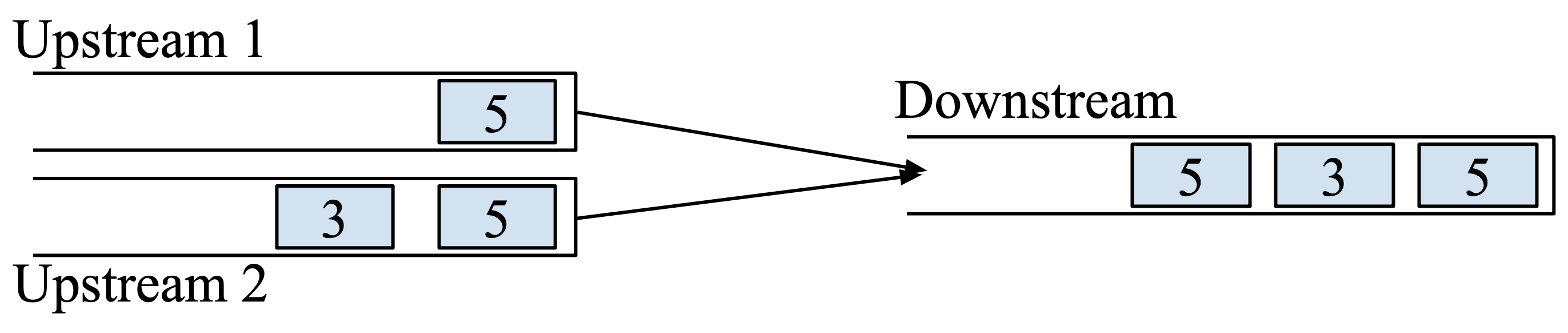## 5.2 Offline diagnosis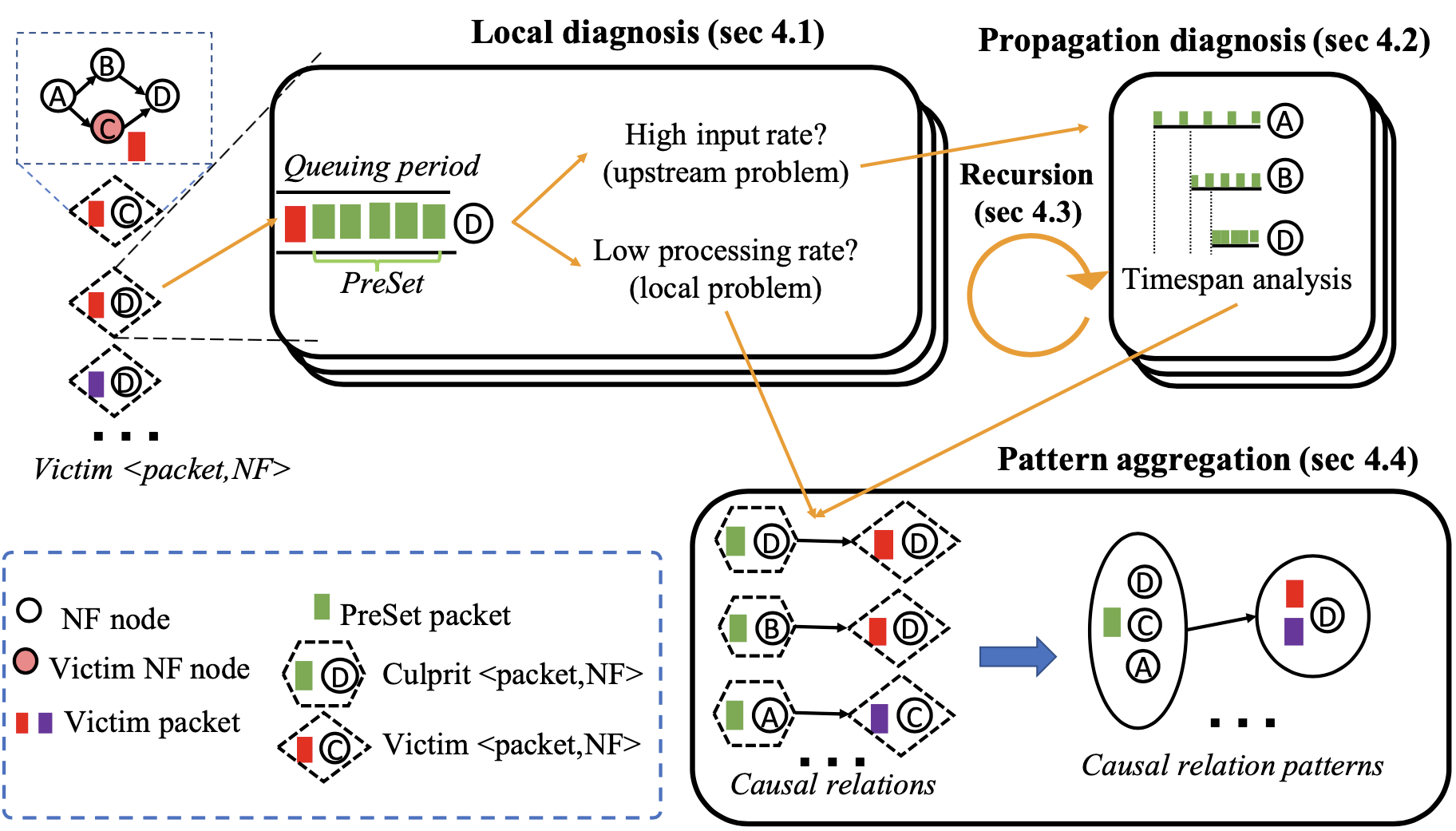# 6. 評估

## 6.1 環境設定

### NF 環境

• 使用 Click-DPDK
• 每個執行個體皆跑在單獨的實體 CPU core 的 VM 中
• 使用 SR-IOV 分配網卡資源
• 使用 MooGen 發送 CAIDA 16 封包
• 使用 64 bytes 大小封包
• 因為 NF 效能表現主要跟封包量有關係，因此該設定能使系統盡量達到較高封包速率

### 拓樸

• 透過 hash 機制做負載平衡到 NAT
• 如果符合特定 Firewall 規則，封包會先被送到 Monitor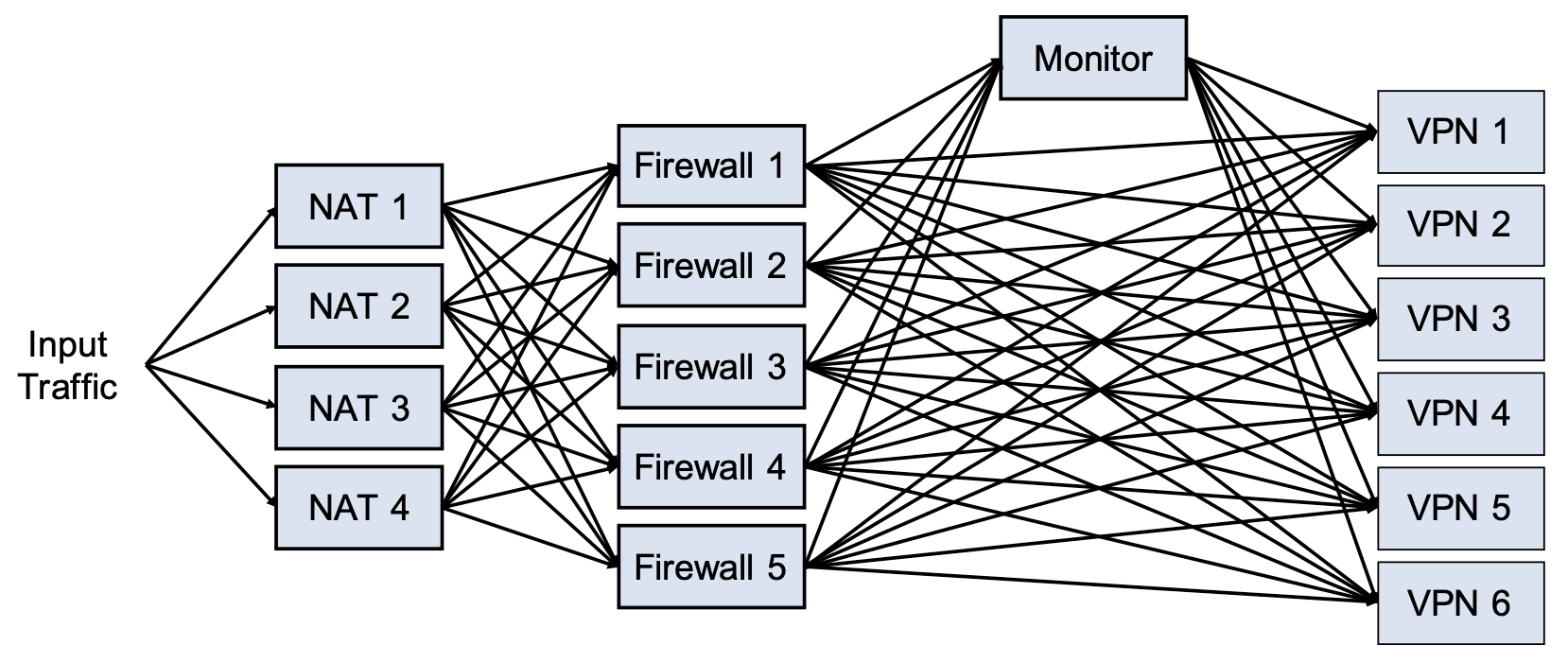### 硬體環境

• Dell R730（MooGen）
• 10 cores, 32 GB RAM
• 2-port 40Gbps Mellanox ConnectX-3 Pro
• Dell T640（16 NFs）
• 2 * 10 cores, 128 GB RAM
• 2-port 40Gbps Mellanox ConnectX-3 Pro

## 6.2 診斷準確性

### 方法

• Traffic burst
• 隨機選一些流發送突發封包，封包量從 500 ~ 2500
• Interrupts
• 隨機挑一個 NF，在 500~100 μs 內給予一個 interrupt
• NF code bugs
• 隨機挑一個 Firewall 注入 bug，其在處理特定流的時候會特別慢（0.05 Mpps）
• 實驗時會注入 50~150 封包量的流來觸發

• 找出 culprit flows（for traffic burst
• 找出 culprit NF（for interrupts
• 找出 culprit NF-flow pairs（for NF code bugs

### 準確性指標

Rank 的順序性依發生原因的可能性排列，也就是 rank 越低代表該 culprit 影響的程度越高，其計算方式是計算某項影響因子對特定元件與對所有相關元件的影響比例的倒數，詳細可參考該論文的 §5.3.3

### 整體準確性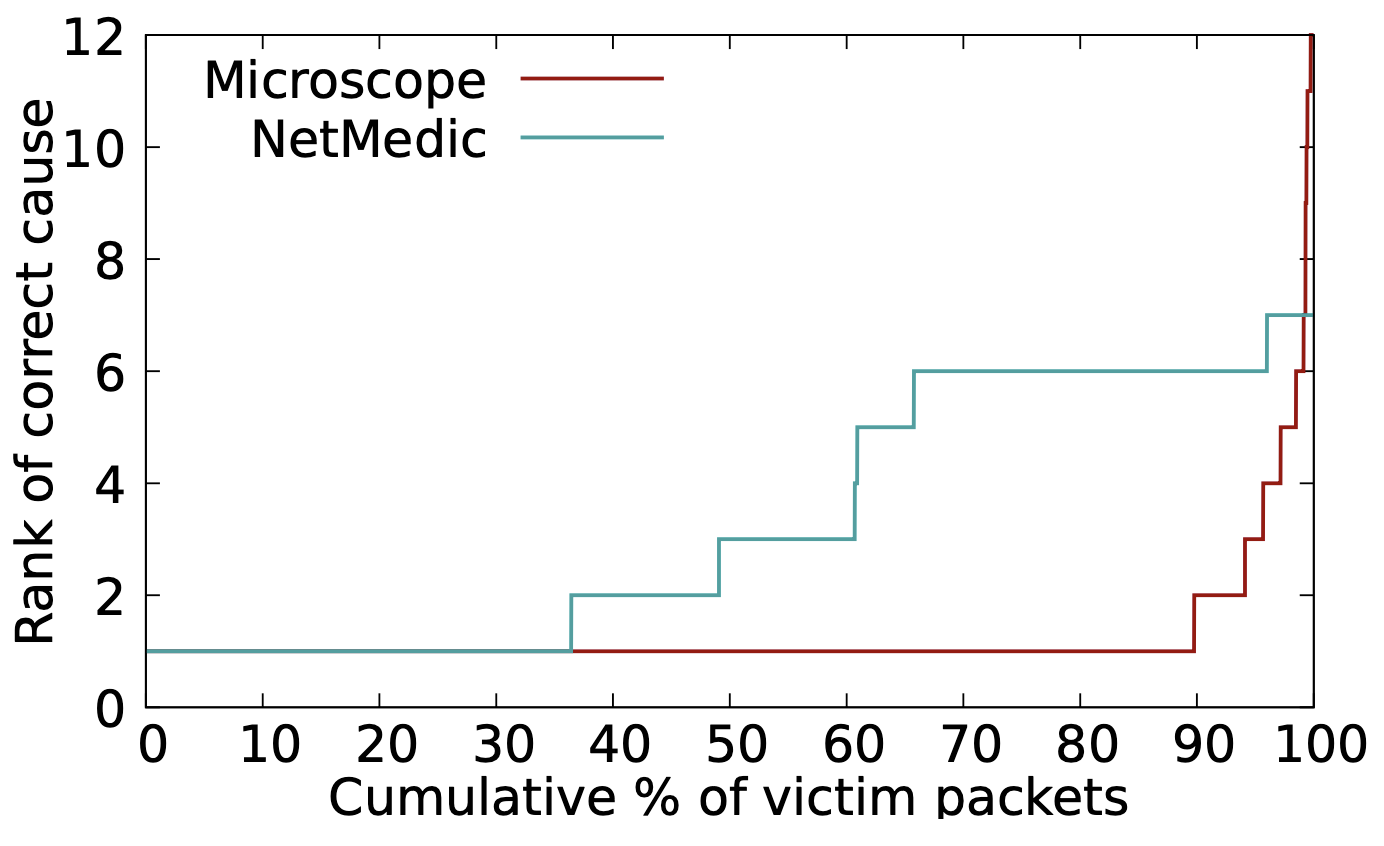### 個別準確性

• 在 interrupt 中
• 有一部分原因是 NetMedic 不太能成功捕獲有時間差的問題，如同 §2 中所描述。
• 在 NF bugs 中
• Microscope 首先歸因於 traffic burst，再來才是 NF bugs。
• NetMedic 正找到 NF bugs 的 culprit 都落於 rank 4~6
• 雖然這是 Firewall 出問題後才影響 VPN，但兩者間有一定的時間差，導致無法正確進行錯誤關聯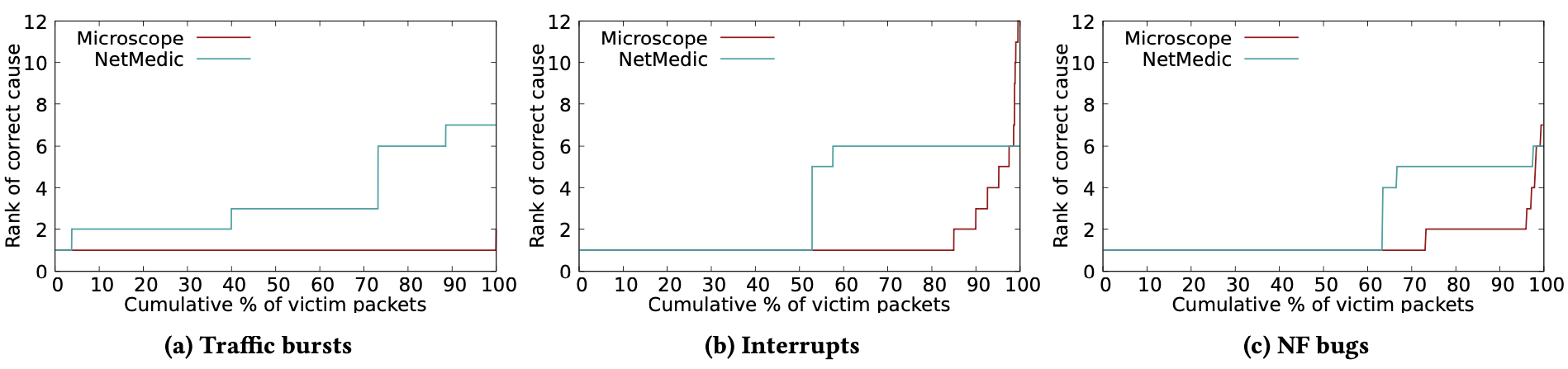### 不同 Time Window Size 對 NetMedic 的影響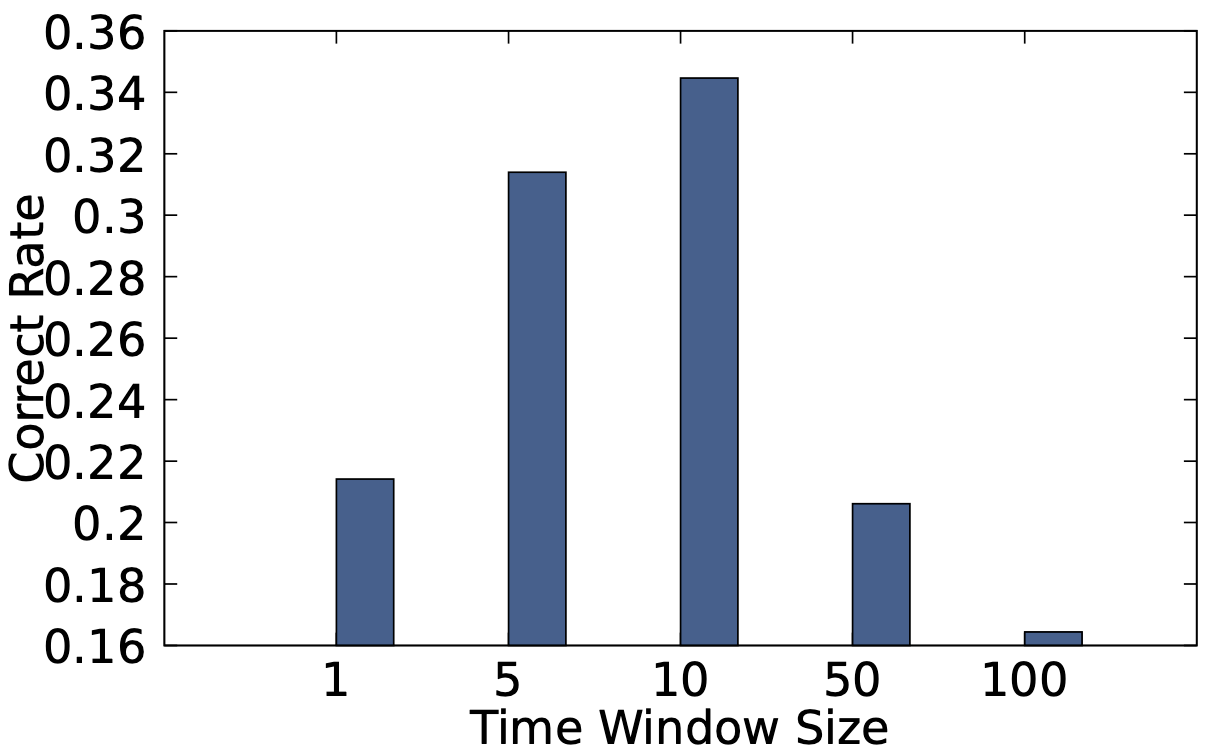## 6.3 詳細評估

• Burst size 的影響
• 使用的 burst size 介於 2000~5000 個封包之間
• 用 5000 封包時，判斷結果最準確
• 隨著封包量下降，準確度下降，因為佇列的長度就變短了
• Interrupt 長度的影響
• 使用長度介於 300 ~ 1500 μs 之間
• 1500 μs 時，判斷結果最準確
• 隨長度下降，準確度下降，因為 buffer 的封包變少了
• Propagation hops 的影響
• 隨著 hop 數提升，準確度下降
• 因為當問題會隨之傳播，使得 victim 也變成 culprit（編按：不確定是否理解正確）

### Culprit 與 Victim NF 間的時間差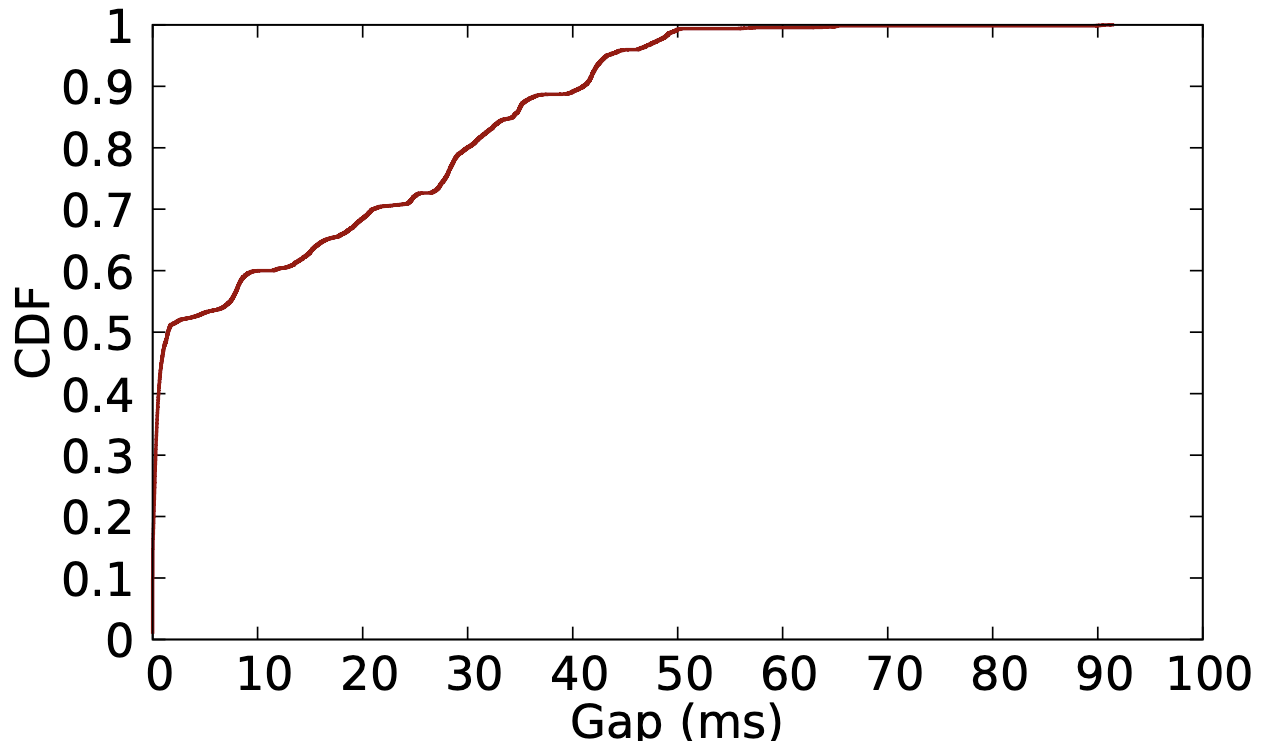# 名詞解釋

## Heavy Hitters (HHs)

### Hierarchical Heavy Hitters (HHHs)

Dimension 可以為 IP prefix, port range, protocol 等等，因此多個 dimension 組成的 HHH 就稱為 multidimensional hierarchical heavy hitters。